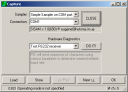Figure 1. Opening Sampler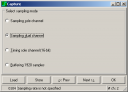Figure 2. Selecting the sampler operational modeFigure 3. Setting sampler optionsFigure 4. Specifying output files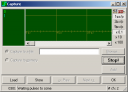Figure 5. Preview started, sampler is waiting for signal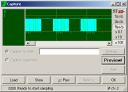Figure 6. Preview data acquiredFigure 7. Ready for sampling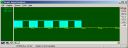Figure 8. Data acquired and visualized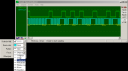Figure 9. Customizing beamsFigure 10. Customizing the surface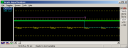Figure 11. Adding a ruler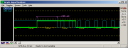Figure 12. Placing a dimestion label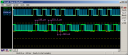Figure 13. Captured another sampleFigure 14. Added yet another ruler